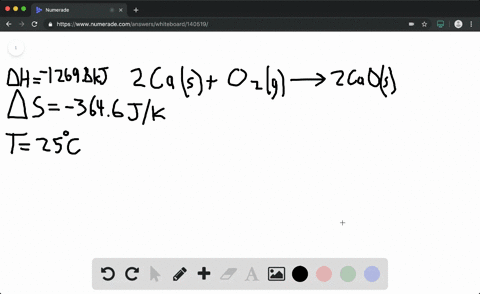### Calculate the free energy change for this reactio…

04:16California State University - East Bay
Problem 45

Calculate the free energy change for this reaction at $25^{\circ} \mathrm{C}$ . Is the
reaction spontaneous? (Assume that all reactants and products
are in their standard states.)
$$\begin{array}{c}{\mathrm{C}_{3} \mathrm{H}_{8}(g)+5 \mathrm{O}_{2}(g) \longrightarrow 3 \mathrm{CO}_{2}(g)+4 \mathrm{H}_{2} \mathrm{O}(g)} \\ {\Delta H_{\mathrm{rxn}}^{\circ}=-2217 \mathrm{kJ} ; \Delta S_{\mathrm{rxn}}^{\circ}=101.1 \mathrm{J} / \mathrm{K}}\end{array}$$

$\Delta G^{\circ}=-2247 \mathrm{kJ}$ and spontaneous

## Discussion

You must be signed in to discuss.

## Video Transcript

Okay, So for this question, we're trying to calculate Delta G, which is free energy. And we wanted also figure whether this reaction is spontaneous honor. To do that, you want to remember this formula? Delta G is equal to Delta H minus T Delta s. I like to call this equation as ghosts because it's kind of resemble goes a little bit because you have g here, right? And the equal to H and the minus sign could be think about, like, negative as an old right. And then we had Delta s time teeth. So th a west are less planning. So to solve this with an anon play and make sure we are watching our unit carefully. So right there, you'll see that it's killed Jules. Right here. You have jewels over Cowan, and I'm right here is our temperature unit, which is in Celsius. So we need to make sure that the unit agreed. Otherwise, we can't continue. Okay, so for this one less plenty. So Delta H is our negative 2 to 17 killer jewels, and we'll keep it there negative. T t is gonna be in Celsius, which you need to change into Calvin to changing Uncover. You're simply adding to 73 then Delta s, which is lie said is one or 1.1. This is Jules per covered, which is gonna be a little bit difference because you have killed Jules on the other one. If the unit Another thing, you can't say track them. So I'm gonna change it into killer Jules by dividing with 1000. So this become point 1011 Okay, so when you saw for this, we're gonna an Afghani equal to negative to hunt 2247. And his unit is gonna be kill jewels. Because Calvin with Assad since Delta jeez, negative. We can say that this reaction is going to be spontaneous. Well,